# Stratified Bayesian Blocks

In 1998, Jeffrey Scargle invented an algorithm to perform optimal binning for photon counting data in gamma-ray observations. He named the algorithm Bayesian Blocks and provided an improved version of it in 2012. It was soon thereafter implemented in Python by Jake Vanderplas with a dynamic programming solution. Vanderplas has since developed a comprehensive implementation of Bayesian Blocks in his astronomy machine learning package astroML.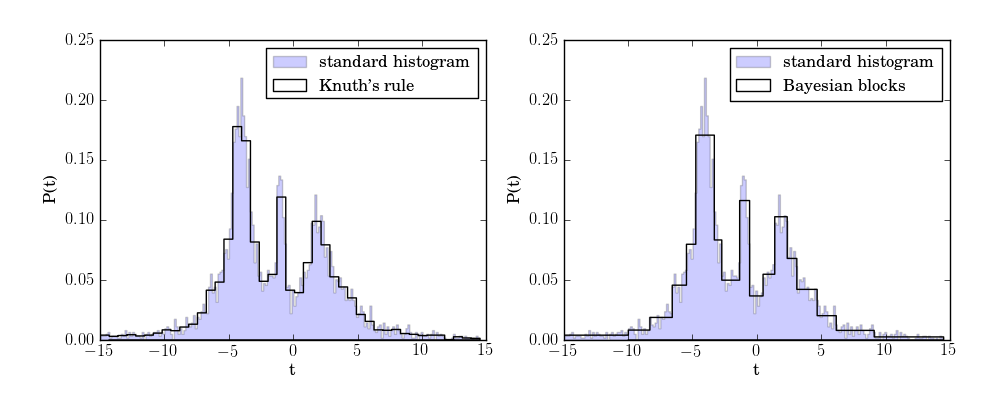Vanderplas illustrates the benefits of Bayesian Blocks in the astroML package

However this implementation suffers from poor performance on one pathological type of data. In particular, data that contain highly-repeated unique values. Although the astroML implementation of Bayesian Blocks addresses this problem for small amounts of repetition, it fails for unique values that have multiplicities on the same order as the total size of the data. Consider the following data which consists of normally distributed unique values that are sampled according to the exponential distribution:

`r = np.random.randn(200)s = np.random.exponential(50, 200).astype(int)data = np.repeat(r, s)`

We’ll abbreviate this normally-distributed, exponentially-sampled distribution as Normal-Exponential data. Running Bayesian Blocks on this data yields the following density estimate.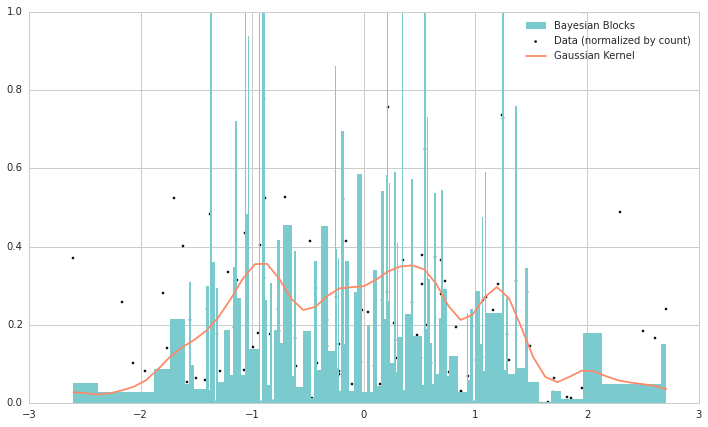Notice how the fine-grained bins make for a very noisy estimate of the underlying probability distribution

In the above, we’ve included a gaussian kernel density estimator (KDE) to illustrate the underlying distribution. Of course, the signal from the histogram bins fails to capture the true underlying distribution. Although gaussian KDE captures the normal distribution in this case, it will incur error when the underlying distribution is less well-behaved.

Our newly-minted Stratified Bayesian Blocks algorithm builds on the existing Bayesian Blocks algorithm to deal with this problem. The first step is to organize the data into strata of repeated values. This can be done by using Bayesian Blocks on the unique value counts themselves.

`t, x = np.unique(data, return_counts = True)r, s = np.unique(x, return_counts=True)s = (np.log(s)+1).astype(int)r = np.repeat(r, s)x_edges = bayesian_blocks(r, p0=0.01)`

Notice the (log(s)+1) regularization of unique value counts above. This helps to reduce the number of strata the algorithm will consider.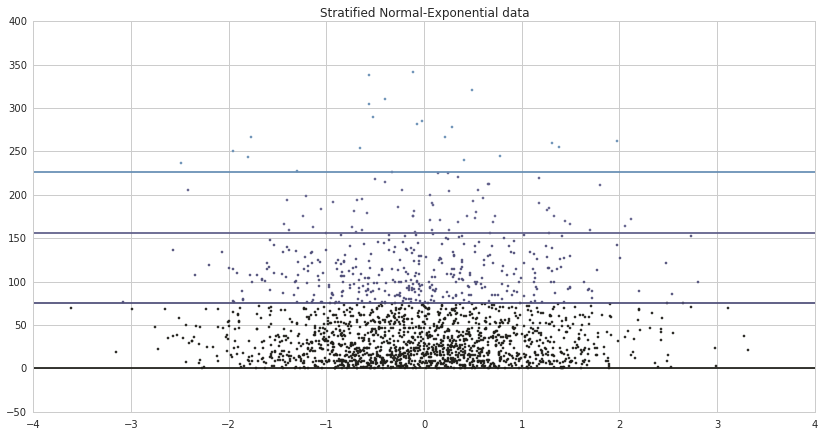Now the data is organized into groups of unique values of similar magnitude

The algorithm now iterates through the strata and performs a round of Bayesian Blocks on each. There are two details that should be noted for this step:

1. The log(s)+1 regularization happens again within each strata. This helps with smoothing
2. At each strata, the unique value count is normalized to the strata’s bottom edge. This ensures that blocks across strata are built in a uniform way.

The result is below: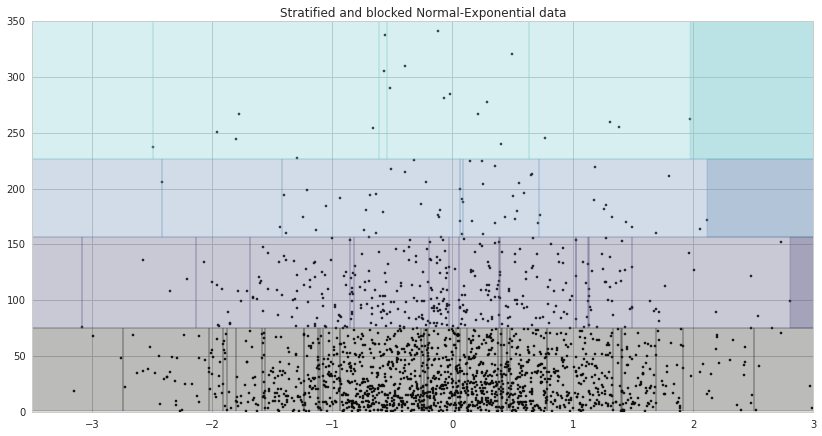Each of the strata now has just a few blocks (this graph is zoomed in a bit)

The only step left is to merge all of the blocks from all of the strata and rebuild the histogram. The (normalized) result is below: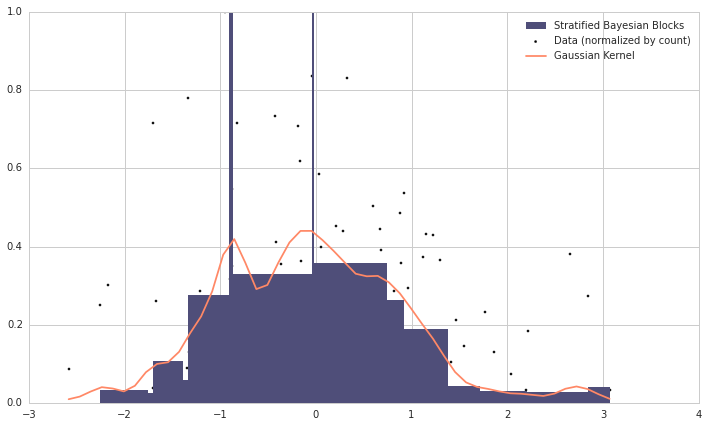The two spikes that appear are artifacts of bin edges from two strata that were close together. They still, however, capture the underlying peaks.

The true benefit of Stratified Bayesian Blocks is evident when we use it to perform an estimate of a posterior distribution. Let’s use as our example, labels constructed from the Normal-Exponential data that are a noisy indicator of the sign (positive or negative) of the data points:

`r = np.random.randn(2000)s = np.random.exponential(50, 2000).astype(int)N = np.sum(s).astype(float)data = np.repeat(r, s)np.random.shuffle(raw)labels = (data > 0).astype(int)label_noise = np.random.choice([0, 1], len(data), p=[0.9, 0.1])labels = np.mod(labels + label_noise, 2)`

Running vanilla Bayesian Blocks on this data yields the graph below

Stratified Bayesian Blocks does notably better in estimating the underlying distribution

It’s difficult to come up with examples that fully illustrate the benefit of Stratified Bayesian Blocks for posterior estimates without using real-world data. Of course, such data is often highly sensitive or proprietary and so we make due with synthetic examples for this post.

Stratified Bayesian Blocks was developed by Jan Florjanczyk and Taylor Sather at ID Analytics in San Diego, CA.

--

--

--

## More from Jan Florjanczyk

Senior Data Scientist @Netflix

Love podcasts or audiobooks? Learn on the go with our new app.## Jan Florjanczyk

Senior Data Scientist @Netflix

## Scikit-learn solvers explained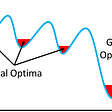## Walking Through Training Models## Feature selection — A look at some of the common techniques